# capi/eeprom.c File Reference

`#include "lpc2xxx.h"`
`#include "eeprom.h"`
`#include "system.h"`
`#include "trace.h"`
Include dependency graph for eeprom.c: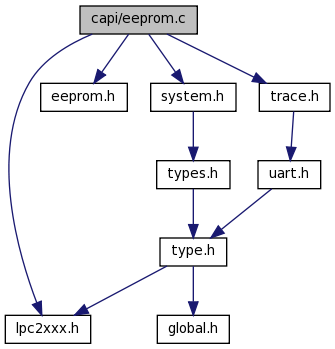## Functions

void init_I2C (void)
int i2c_stop ()
unsigned char i2c_tx (int send_start, int send_stop, unsigned char byte)
unsigned char i2c_rx (int nak, int send_stop)
unsigned char start_I2C_EE_write (unsigned int address)
unsigned char read_I2C_EE (unsigned int address, unsigned char *buff, unsigned int num)
unsigned char read_I2C_EE_stream (unsigned long address, unsigned long num, funcPutByte fpb, unsigned long userdata, unsigned long *pChksum)
unsigned int write_I2C_EE_stream (unsigned int address, funcGetByte fgb, unsigned int num, unsigned long *chkAdd)
unsigned int write_I2C_EE (unsigned int address, unsigned char *buff, unsigned int num)
unsigned int write_I2C_EE_byte (unsigned int address, unsigned char b)

## Variables

unsigned char g_i2c_init = 0
unsigned char g_i2c_start = 0
unsigned int I2CSPEED = 6

## Function Documentation

 unsigned char i2c_rx ( int nak, int send_stop )

Here is the caller graph for this function: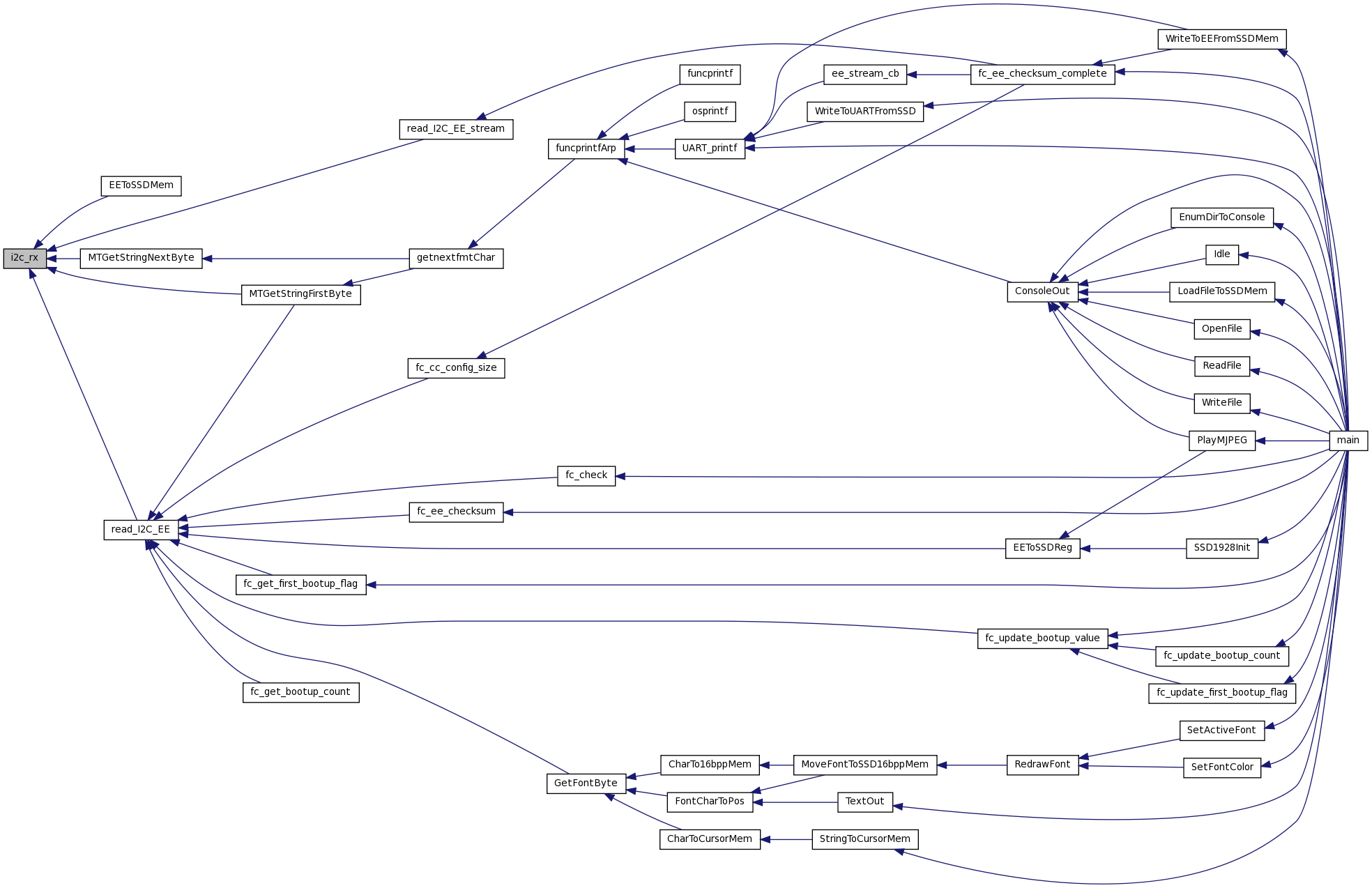int i2c_stop ( )
 unsigned char i2c_tx ( int send_start, int send_stop, unsigned char byte )

Here is the caller graph for this function:void init_I2C ( void )

References g_i2c_init, I2CSCL1, I2CSCLOUT, I2CSDA1, I2CSDAOUT, PINSEL0, PINSEL1, TRACE, and TRACE1.

Here is the caller graph for this function:unsigned char read_I2C_EE ( unsigned int address, unsigned char * buff, unsigned int num )

References i2c_rx(), i2c_tx(), init_I2C(), and start_I2C_EE_write().

Here is the call graph for this function:Here is the caller graph for this function:unsigned char read_I2C_EE_stream ( unsigned long address, unsigned long num, funcPutByte fpb, unsigned long userdata, unsigned long * pChksum )

References i2c_rx(), i2c_tx(), init_I2C(), and start_I2C_EE_write().

Referenced by fc_ee_checksum_complete().

Here is the call graph for this function: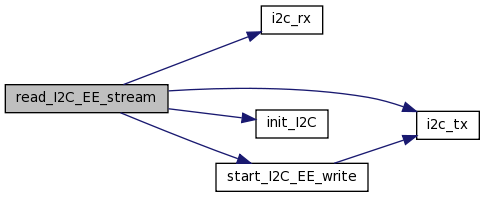Here is the caller graph for this function:unsigned char start_I2C_EE_write ( unsigned int address )

References i2c_tx().

Here is the call graph for this function:Here is the caller graph for this function:unsigned int write_I2C_EE ( unsigned int address, unsigned char * buff, unsigned int num )

References i2c_tx(), I2CSPEED, init_I2C(), and start_I2C_EE_write().

Here is the call graph for this function:unsigned int write_I2C_EE_byte ( unsigned int address, unsigned char b )

References i2c_tx(), I2CSPEED, init_I2C(), and start_I2C_EE_write().

Referenced by fc_update_bootup_value(), and WriteToEEFromSSDMem().

Here is the call graph for this function: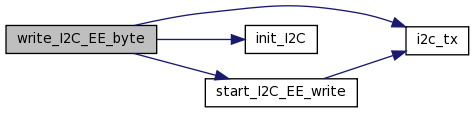Here is the caller graph for this function: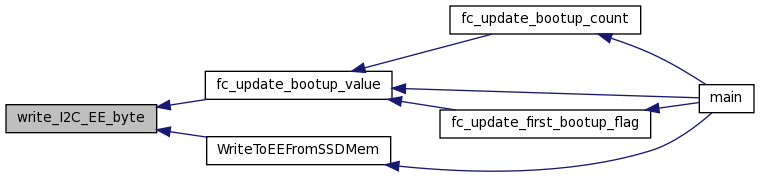unsigned int write_I2C_EE_stream ( unsigned int address, funcGetByte fgb, unsigned int num, unsigned long * chkAdd )

References delay_ms(), i2c_tx(), I2CSPEED, init_I2C(), and start_I2C_EE_write().

Here is the call graph for this function:## Variable Documentation

 unsigned char g_i2c_init = 0

Referenced by init_I2C().

 unsigned char g_i2c_start = 0
 unsigned int I2CSPEED = 6
Generated on Wed Mar 23 11:08:28 2011 for Omnima LCD by1.6.3